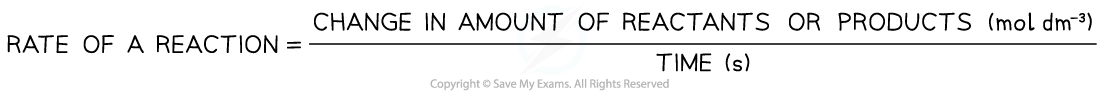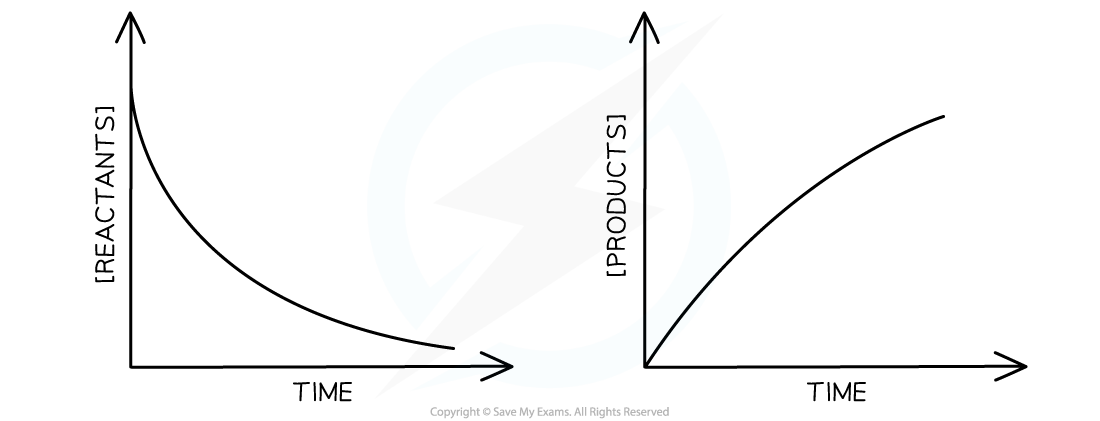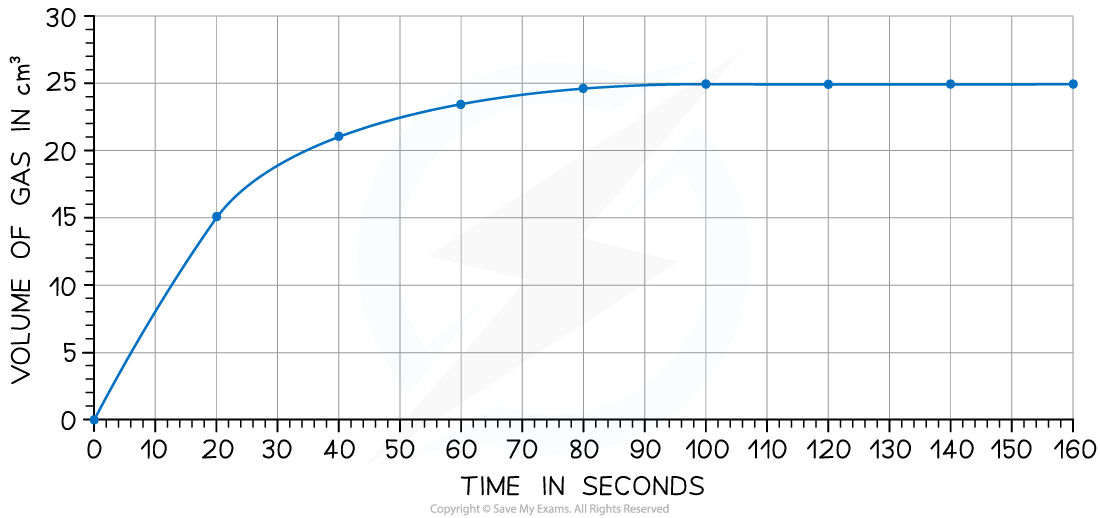# IB DP Chemistry: SL复习笔记6.1.2 Rate of Reaction

### Rate of Reaction

#### Reaction rate

• Some reactions take place instantly, but most are much slower and it is possible to measure how long these reactions take to reach a certain stage
• As a chemical reaction proceeds, the concentration of the reactants decreases and the concentration of the products increases
• The rate of a reaction is the speed at which a chemical reaction takes place and has units mol dm-3 s-1
• The rate of a reaction can be calculated by:• Graphically we can represent the rate of reaction as:Rate of reaction graphs

#### Worked Example

Iodine and methanoic acid react in aqueous solution.

I2 (aq) + HCOOH (aq) → 2I (aq) + 2H+ (aq) + CO2 (g)

The rate of reaction can be found by measuring the volume of carbon dioxide produced per unit time and plotting a graph as shownCalculate the rate of reaction at 20 seconds

• Draw a tangent to the curve at 20 seconds:• Complete the triangle and read off the values of x and y
• Determine the gradient of the line using ∆y / ∆x
• Rate of reaction = 24 ÷ 40 = 0.60 cms-1

#### Exam Tip

When drawing the tangent to a curve make the triangle large and try to intersect with gridlines if you can. This minimises errors of precision and reduces the chance you will accidently misread the graph values